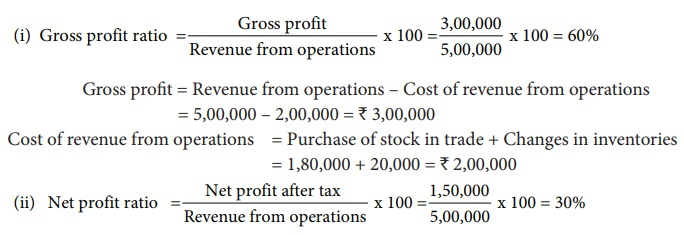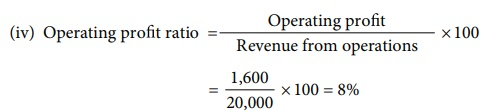Home | | Accountancy 12th Std | Profitability ratios

# Profitability ratios

Profitability ratios help to assess the profitability of a business concern. These ratios also help to analyse the earning capacity of the business in terms of utilisation of resources employed in the business.

Profitability ratios

Profitability ratios help to assess the profitability of a business concern. These ratios also help to analyse the earning capacity of the business in terms of utilisation of resources employed in the business. Generally these ratios are expressed as a percentage.

The profitability ratios commonly used are

(i) Gross profit ratio

(ii) Operating cost ratio

(iii) Operating profit ratio

(iv) Net profit ratio

(v) Return on investment

## (i) Gross profit ratio

Gross profit ratio is the proportion of gross profit to net revenue from operations. Gross profit ratio shows the margin of profit available out of revenue from operations. It is computed as below:Gross profit ratio = [ Gross profit / Revenue from operations ] × 100

Gross profit = Revenue from operations – Cost of revenue from operations

A higher gross profit ratio indicates high profitability. It should be sufficiently high to provide for indirect expenses to be paid by a business.

Illustration 13

Calculate gross profit ratio from the following:

Revenue from operations 1,00,000, Cost of revenue from operations 80,000 and purchases 62,500.

SolutionGross profit = Revenue from operations – Cost of revenue from operations

= 1,00,000 – 80,000 = ₹ 20,000

## (ii) Operating cost ratio

Operating cost ratio is the proportion of operating cost to revenue from operations. This ratio is a test of the operational efficiency of the business. It is calculated as under.Operating cost ratio = [ Operating cost / Revenue from operations ] × 100

Operating cost is the cost which is associated with the operating activities of the business.

Operating cost = Cost of revenue from operations + Operating expenses

Operating expenses = Employee benefit expenses + Depreciation + Other expenses related to office and administration, selling and distribution

A lower operating ratio indicates better profitability. Lesser the operating cost ratio, higher is the margin available for payment of non operating expenses such as interest on loans, loss on sale of fixed assets, etc.

Illustration 14

Following is the statement of profit and loss of Maria Ltd. for the year ended 31st March, 2018.

Calculate the operating cost ratio.SolutionOperating cost ratio = [ Operating cost / Revenue from operations ] × 100

= 4,80,000 /8,00,000 × 100 = 60%

Cost of revenue from operations = Purchases of stock-in-trade + Change in inventories of stock in trade + Direct expenses (wages) = 4,50,000 + (40,000) + 10,000 = 4,20,000

Operating expenses = Administrative expenses+Selling         and    distributionexpenses+ Employee benefits expenses (salaries) = 20,000 + 28,000 + 12,000 = 60,000

Operating cost = Cost of revenue from operations + Operating expenses

= 4,20,000 + 60,000= 4,80,000

Tutorial Note

Loss on sale of fixed assets is a non-operating item, hence it is ignored.

## (iii) Operating profit ratio

Operating profit ratio gives the proportion of operating profit to revenue from operations. Operating profit ratio is an indicator of operational efficiency of an organisation. It may be computed as follows:Operating profit ratio = [ Operating profit x Revenue from operations ] × 100

Alternatively, it is calculated as under.

Operating profit ratio = 100 – Operating cost ratio

Operating profit   = Revenue from operations – Operating cost

A higher ratio indicates better profitability. Greater the operating ratio, higher is the margin available for paying non-operating expenses.

Tutorial note

Operating cost ratio + Operating profit ratio = 100%

Illustration 15

Calculate operating profit ratio under the following cases.

Case 1: Revenue from operations 10,00,000, Operating profit 1,50,000.

Case 2: Revenue from operations 15,00,000, Operating cost 12,00,000.

Case 3: Revenue from operations 20,00,000, Gross profit 30% on revenue from operations, Operating expenses 4,00,000

Solution## (iv) Net profit ratio

Net profit ratio is the percentage of net profit on revenue from operations. It is calculated as under:Net profit ratio = [ Net profit after tax / Revenue from operations ] × 100

Net profit after tax = Gross profit + Indirect income – Indirect expenses – Tax (OR)

Net profit after tax = Revenue from operations – Cost of revenue from operations – Operating expenses –Non operating expenses + Non-operating income - Tax

Net profit ratio is an indicator of the overall profitability of the business. A higher net profit ratio indicates high profitability.

Illustration 16

From the following details of a business concern calculate net profit ratio.SolutionNet profit = Revenue from operations – Cost of revenue from operations – Administration expenses – Selling expenses = 3,50,000 – 1,50,000 – 50,000 – 10,000 = 1,40,000

Tutorial note

It is assumed that there is no tax payable.

Illustration 17

From the following statement of profit and loss of Mukesh Ltd. Calculate

(i) Gross profit ratio  (ii) Net profit ratio.SolutionIllustration 18

From the following trading activities of Naveen Ltd. calculate

(i) Gross profit ratio (ii) Net profit ratio (iii) Operating cost ratio (iv) Operating profit ratioSolutionCost of revenue from operations = Purchase of stock-in-trade + Changes in inventory + Direct expenses

= 17,000 – 1,000 + 0 = ₹ 16,000

Gross profit = Revenue from operations – Cost of revenue from operations

= 20,000 – 16,000 = ₹ 4,000Tutorial note

It is assumed that there is no tax payable.Operating cost = Cost of revenue from operations + Operating expenses

Operating expenses = Other expenses = ₹ 2,400

Operating cost = 16,000 + 2,400 = ₹ 18,400Operating profit = Revenue from operations – Operating cost

= 20,000 – 18,400 = ₹ 1,600

## (v) Return on Investment (ROI)

Return on investment shows the proportion of net profit before interest and tax to capital employed (shareholders’ funds and long term debts). This ratio measures how efficiently the capital employed is used in the business. It is an overall measure of profitability of a business concern. It is computed as below:Return on Investment (ROI) = [ Net profit before interest and tax / Capital employed ] x 100

Capital employed = Shareholders’ funds + Non current liabilities

Greater the return on investment better is the profitability of a business and vice versa.

Illustration 19

Following is the extract of the balance sheet of Babu Ltd., as on 31st March, 2018:Net profit before interest and tax for the year was ₹ 25,000. Calculate the return on capital employed for the year.

SolutionCapital employed = Share capital + Reserves and surplus + Long term borrowings

= 70,000 + 25,000 +30,000 = ₹ 1,25,000

Tags : Computation of ratios | Accountancy , 12th Accountancy : Chapter 9 : Ratio Analysis
Study Material, Lecturing Notes, Assignment, Reference, Wiki description explanation, brief detail
12th Accountancy : Chapter 9 : Ratio Analysis : Profitability ratios | Computation of ratios | Accountancy